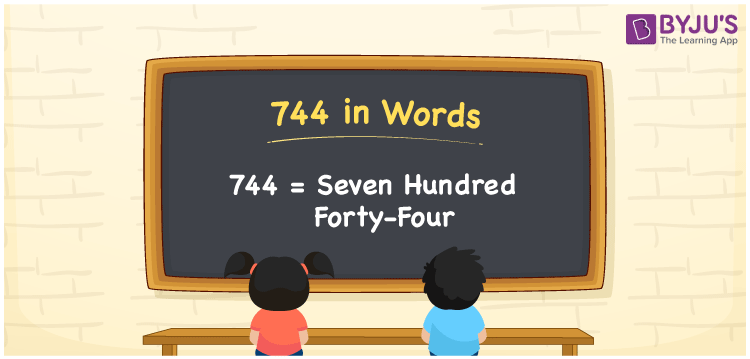# 744 in Words

We can write 744 in words as Seven hundred forty-four. If you bought a box of pens at Rs. 744, then you can say, “I bought a box of pens for Seven hundred forty-four rupees”. In this article, you will understand how to convert the cardinal number 744 into words with the help of a place value chart.

 744 in words Seven hundred forty-four Seven hundred forty-four in Numbers 744

## 744 in English Words

We generally use the English alphabet to express numbers in words. So, we spell 744 in English words as “Seven hundred forty-four”.## How to Write 744 in Words?

The number 744 contains three digits. Thus, we need to create a place value chart with three columns, as shown below.

 Hundreds Tens Ones 7 4 4

Here, ones = 4, tens = 4, hundreds = 7

So, 7 × Hundred + 4 × Ten + 4 × One

= 7 × 100 + 4 × 10 + 4 × 1

= 700 + 40 + 4

= Seven hundred + Forty + Four

= Seven hundred forty-four

Therefore, 744 in words is written as Seven hundred forty-four.

As we know, 744 is a natural number that precedes 745 and succeeds 743.

744 in words – Seven hundred forty-four

Is 744 an odd number? – No

Is 744 an even number? – Yes

Is 744 a prime number? – No

Is 744 a composite number? – Yes

Is 744 a perfect square number? – No

Is 744 a perfect cube number? – No

## Frequently Asked Questions on 744 in Words

Q1

### Write the number 744 in words.

We can write the number 744 in English words as Seven hundred forty-four.
Q2

### Express the value of 744 + 900 in words.

744 + 900 = 1644 Thus, the value of 744 + 900, i.e., 1644 can be expressed as One thousand six hundred forty-four.
Q3

### How to write Rs. 744 in words on a cheque?

On a cheque, we generally write Rs. 744 in words as “Seven hundred forty-four rupees only”.